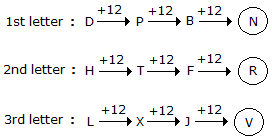Courses

# Test: Letter Series Type 2

## 30 Questions MCQ Test UPSC CSAT Preparation | Test: Letter Series Type 2

Description
This mock test of Test: Letter Series Type 2 for UPSC helps you for every UPSC entrance exam. This contains 30 Multiple Choice Questions for UPSC Test: Letter Series Type 2 (mcq) to study with solutions a complete question bank. The solved questions answers in this Test: Letter Series Type 2 quiz give you a good mix of easy questions and tough questions. UPSC students definitely take this Test: Letter Series Type 2 exercise for a better result in the exam. You can find other Test: Letter Series Type 2 extra questions, long questions & short questions for UPSC on EduRev as well by searching above.
QUESTION: 1

### Directions to Solve In each of the following questions, various terms of an alphabet series are given with one or more terms missing as shown by (?). Choose the missing terms out of the given alternatives. Question - WFB, TGD, QHG, ?

Solution: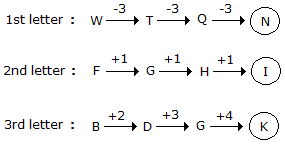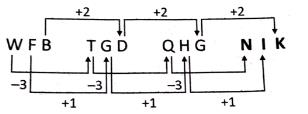QUESTION: 2

### Directions to Solve In each of the following questions, various terms of an alphabet series are given with one or more terms missing as shown by (?). Choose the missing terms out of the given alternatives. Question - AZ, GT, MN, ?, YB

Solution: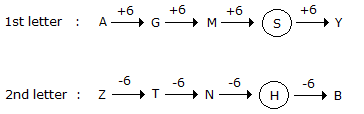QUESTION: 3

### Directions to Solve In each of the following questions, various terms of an alphabet series are given with one or more terms missing as shown by (?). Choose the missing terms out of the given alternatives. Question - H, I, K, N, ?

Solution: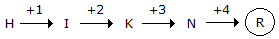QUESTION: 4

Directions to Solve

In each of the following questions, various terms of an alphabet series are given with one or more terms missing as shown by (?). Choose the missing terms out of the given alternatives.

Question -

Z, S, W, O, T, K, Q, G, ?, ?

Solution:

The given sequence is a combination of two series:

I. Z, W, T, Q, ? and II. S, O, k, G, ?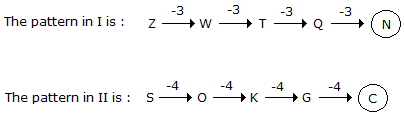QUESTION: 5

Directions to Solve

In each of the following questions, various terms of an alphabet series are given with one or more terms missing as shown by (?). Choose the missing terms out of the given alternatives.

Question -

b e d f ? h j ? l

Solution:

The series may be divided into groups as shown:

b e d / f ? h / j ? l

Clearly in the first group, the second and third letters are respectively three and two steps ahead of the first letter.

A similar pattern would follow in the second and third groups.

QUESTION: 6

Directions to Solve

In each of the following questions, various terms of an alphabet series are given with one or more terms missing as shown by (?). Choose the missing terms out of the given alternatives.

Question -

A, G, L, P, S, ?

Solution: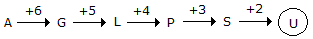QUESTION: 7

Directions to Solve

In each of the following questions, various terms of an alphabet series are given with one or more terms missing as shown by (?). Choose the missing terms out of the given alternatives.

Question -

ajs, gpy, ?, sbk, yhq

Solution: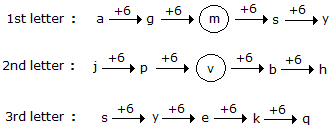QUESTION: 8

Directions to Solve

In each of the following questions, various terms of an alphabet series are given with one or more terms missing as shown by (?). Choose the missing terms out of the given alternatives.

Question -

Y, B, T, G, O, ?

Solution:

The given sequence is a combination of two series :

I. Y,T, O and II. B, G, ?

I consists of 2nd, 7th and 12th letters from the end of the English alphabet, while

II consists of 2nd, 7th and 12th letters from the beginning of the English alphabet.

So, the missing letter in II is the 12th letter from the beginning of the English alphabet, which is L.

QUESTION: 9

Directions to Solve

In each of the following questions, various terms of an alphabet series are given with one or more terms missing as shown by (?). Choose the missing terms out of the given alternatives.

Question -

AB, DEF, HIJK, ?, STUVWX

Solution:

The number of letters in the terms of the given series increases by one at each step.

The first letter of each term is two steps ahead of the last letter of the preceding term.

However, each term consists of consecutive letters in order.

QUESTION: 10

Directions to Solve

In each of the following questions, various terms of an alphabet series are given with one or more terms missing as shown by (?). Choose the missing terms out of the given alternatives.

Question -

C, Z, F, X, I, V, L, T, O, ?, ?

Solution:

The given sequence is a combination of two series:

I. C, F, I, L, O, ? and II. Z, X, V, T, ?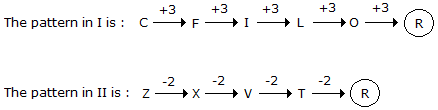QUESTION: 11

Directions to Solve

In each of the following questions, various terms of an alphabet series are given with one or more terms missing as shown by (?). Choose the missing terms out of the given alternatives.

Question -

A, B, B, D, C, F, D; H, E, ?, ?

Solution:

The given sequence is a combination of two series :

I. 1st, 3rd, 5th, 7th, 9th, 11th terms i.e. A, B, C, D, E, ? II. 2nd, 4th, 6th, 8th, 10th terms i.e. B, D, F, H, ?

Clearly, I consists of consecutive letters while II consists of alternate letters. So, the missing letter in I is F, while that in II is J. So, the missing terms i.e. 10th and 11th terms are J and F respectively.

QUESTION: 12

Directions to Solve

In each of the following questions, various terms of an alphabet series are given with one or more terms missing as shown by (?). Choose the missing terms out of the given alternatives.

Question -

Z, X, S, I, R, R, ?, ?

Solution: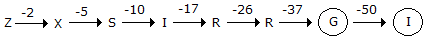Note that the numbers representing the difference between the consecutive terms of the series again from a series - 2, 5, 10, 17, 26, 37, 50 - in which the pattern is +3, +5, +7, +9, +11, +13.

QUESTION: 13

Directions to Solve

In each of the following questions, various terms of an alphabet series are given with one or more terms missing as shown by (?). Choose the missing terms out of the given alternatives.

Question -

T, R, P, N, L, ?, ?

Solution: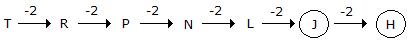QUESTION: 14

Directions to Solve

In each of the following questions, various terms of an alphabet series are given with one or more terms missing as shown by (?). Choose the missing terms out of the given alternatives.

Question -

AYBZC, DWEXF, GUHVI, JSKTL, ?

Solution: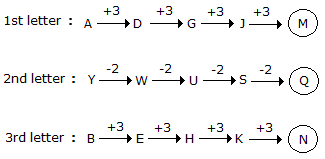QUESTION: 15

Directions to Solve

In each of the following questions, various terms of an alphabet series are given with one or more terms missing as shown by (?). Choose the missing terms out of the given alternatives.

Question -

PMT, OOS, NQR, MSQ, ?

Solution: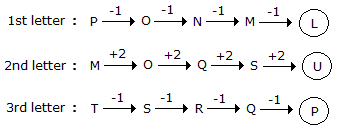QUESTION: 16

Directions to Solve

In each of the following questions, various terms of an alphabet series are given with one or more terms missing as shown by (?). Choose the missing terms out of the given alternatives.

Question -

A, B, N, C, D, O, E, F, P, ?, ?, ?

Solution:

The given series may be divided into 2 groups :

I. A, B, C, D, E, F, ?, ? and II. N, O, P, ?

Clearly, the given series consists of two terms of I followed by one term of II.

The missing terms in I are G and H while the missing term in II is Q.

QUESTION: 17

Directions to Solve

In each of the following questions, various terms of an alphabet series are given with one or more terms missing as shown by (?). Choose the missing terms out of the given alternatives.

Question -

GH, JL, NQ, SW, YD, ?

Solution: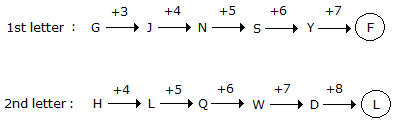QUESTION: 18

Directions to Solve

In each of the following questions, various terms of an alphabet series are given with one or more terms missing as shown by (?). Choose the missing terms out of the given alternatives.

Question -

R, U, X, A, D, ?

Solution: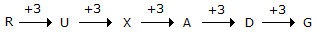QUESTION: 19

Directions to Solve

In each of the following questions, various terms of an alphabet series are given with one or more terms missing as shown by (?). Choose the missing terms out of the given alternatives.

Question -

AYD, BVF, DRH, ?, KGL

Solution: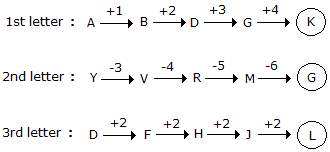QUESTION: 20

Directions to Solve

In each of the following questions, various terms of an alphabet series are given with one or more terms missing as shown by (?). Choose the missing terms out of the given alternatives.

Question -

AZ, CX, FU, ?

Solution: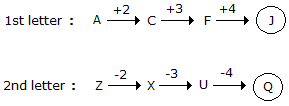QUESTION: 21

Directions to Solve

In each of the following questions, various terms of an alphabet series are given with one or more terms missing as shown by (?). Choose the missing terms out of the given alternatives.

Question -

Solution:

One letter from the beginning and one from the end of a term are removed, one by one, in alternate steps.

QUESTION: 22

Directions to Solve

In each of the following questions, various terms of an alphabet series are given with one or more terms missing as shown by (?). Choose the missing terms out of the given alternatives.

Question -

A, D, H, M, ?, Z

Solution: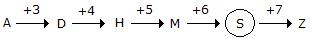QUESTION: 23

Directions to Solve

In each of the following questions, various terms of an alphabet series are given with one or more terms missing as shown by (?). Choose the missing terms out of the given alternatives.

Question -

PERPENDICULAR, ERPENDICULA, RPENDICUL, ?

Solution:

Each term of the series is obtained by removing two letters from the preceding term one from the beginning and one from the end, So, the missing term is PENDICU.

QUESTION: 24

Directions to Solve

In each of the following questions, various terms of an alphabet series are given with one or more terms missing as shown by (?). Choose the missing terms out of the given alternatives.

Question -

Z, ?, T, ?, N, ?, H, ?, B

Solution: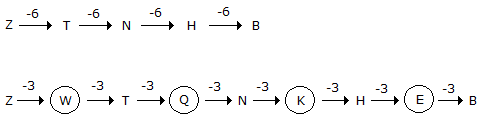QUESTION: 25

Directions to Solve

In each of the following questions, various terms of an alphabet series are given with one or more terms missing as shown by (?). Choose the missing terms out of the given alternatives.

Question -

ejo tyd ins xch ?

Solution:

There is a gap of four letters between the first and second, the second and third letters of each term, and also between the last letter of a term and the first letter of the next term.

QUESTION: 26

Directions to Solve

In each of the following questions, various terms of an alphabet series are given with one or more terms missing as shown by (?). Choose the missing terms out of the given alternatives.

Question -

Z, U, Q, ?, L

Solution: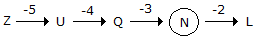QUESTION: 27

Directions to Solve

In each of the following questions, various terms of an alphabet series are given with one or more terms missing as shown by (?). Choose the missing terms out of the given alternatives.

Question -

M, N, O, L, R, I, V, ?

Solution:

The given sequence is a combination of two series:

I. M, O, R, V and II. N, L, I, ?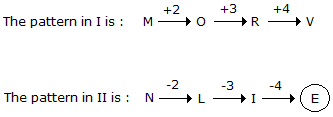QUESTION: 28

Directions to Solve

In each of the following questions, various terms of an alphabet series are given with one or more terms missing as shown by (?). Choose the missing terms out of the given alternatives.

Question -

Solution:

In the first step, one letter from the beginning and one from the end of a term are removed to give the next term. In the second step, two letters from the beginning of a term are removed.

These two steps are repeated alternately.

QUESTION: 29

Directions to Solve

In each of the following questions, various terms of an alphabet series are given with one or more terms missing as shown by (?). Choose the missing terms out of the given alternatives.

Question -

B, D, F, I, L, P, ?

Solution: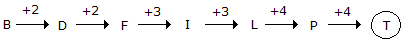QUESTION: 30

Directions to Solve

In each of the following questions, various terms of an alphabet series are given with one or more terms missing as shown by (?). Choose the missing terms out of the given alternatives.

Question -

DHL, PTX, BFJ, ?

Solution: